• 1. 对角矩阵参与矩阵乘法 矩阵 A 左乘一个对角矩阵 D，是分别用 D 的对角线元素分别作用于矩阵 A 的每一行； 相似地，矩阵 A 右乘一个对角矩阵 D，是分别将 D 的对角线元素分别作用于矩阵 A 的每一列 对角矩阵之间的...
1. 对角矩阵参与矩阵乘法

矩阵 A 左乘一个对角矩阵 D，是分别用 D 的对角线元素分别作用于矩阵 A 的每一行；
相似地，矩阵 A 右乘一个对角矩阵 D，是分别将 D 的对角线元素分别作用于矩阵 A 的每一列
对角矩阵之间的矩阵乘法运算，对角线元素相乘，仍为对角矩阵，自然此时满足乘法的交换律；

展开全文• ## 矩阵对角化

万次阅读 2015-10-16 16:52:47
一、矩阵对角化的理论 一个映射或者一个线性变换，都有一个矩阵和它相对应。矩阵或者映射是不是可以对角化，对工程应用来说比较重要，因为对角化后的矩阵，乘积简单，经过多次变换的话，相当于矩阵的多次方。矩阵...
一、矩阵对角化的理论
一个映射或者一个线性变换，都有一个矩阵和它相对应。矩阵或者映射是不是可以对角化，对工程应用来说比较重要，因为对角化后的矩阵，乘积简单，经过多次变换的话，相当于矩阵的多次方。矩阵能不能对角化，取决于它的特称向量能否构成矩阵的一个基。
1．在域 F 上的 n × n 矩阵 A 是可对角化的，当且仅当它的特征空间的维度等于 n，它为真当且仅当存在由 A 的特征向量组成的Fn的基。如果找到了这样的基，可以形成有基向量作为纵列的矩阵 P，而 P-1AP 将是对角矩阵。这个矩阵的对角元素是 A 的特征值。
2. 线性映射 T : V → V 是可对角化的，当且仅当它的特征空间的维度等于 dim(V)，它为真当且仅当存在由 T 的特征向量组成的 V 的基。T 关于这个基将表示为对角矩阵。这个矩阵的对角元素是 T 的特征值。
另一个特征化: 矩阵或线性映射在域 F 上可对角化的，当且仅当它的极小多项式在 F 上有不同的线性因子。

下列充分(但非必要)条件经常是有用的。
1.  n × n 矩阵 A 只在域 F 上可对角化的，如果它在 F 中有 n 个不同的特征值，就是说，如果它的特征多项式在 F 中有 n 个不同的根。
2.  线性映射 T : V → V 带有 n=dim(V) 是可对角化的，如果它有 n 个不同的特征值，就是说它的特征多项式在 F 中有 n 个不同的根。
3. 在域 F 上的 n × n 矩阵 A，如果重根的维数等于其线性无关的特征向量的个数，则矩阵A可以对角化。
4.
二、矩阵对角化过程
下面例子中，矩阵A是对称的，它可以进行对角化，虽然它只有2个不同的特征值，但是有4个线性无关的特征向量，所以能进行对角化。
给定矩阵A，求它的特征值、特征向量，并对它进行对角化。
（1）求特征多项式，matlab命令p= poly(A)；
（2）求解特征多项式，求出特征值，solve（P）；
（3）分别将特征值带入齐次方程组，求出基础解系
（4）针对每个特征值下的基础解系，进行正交化。
（5）对正交化后的向量单位化

例子：已知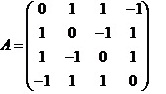求出一正交矩阵 使 成对角形.
解：先求出的 特征值.由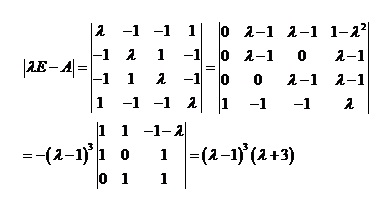即得的特征值为 （三重）， .
其次，求属于1的特征向量.把 代入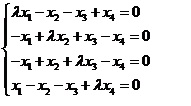（*）
求得基础解系为

把它们正交化，得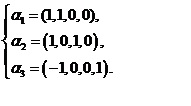再单位化，得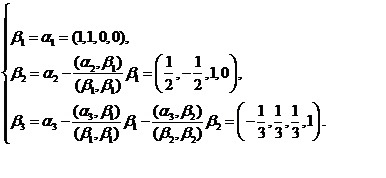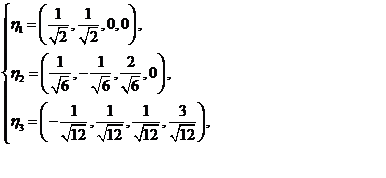这是属于三重特征值 三个标准正交的特征向量.
再求属于 的特征向量.用代入（*）式求得其基础解系为 .
把它单位化，得.
特征向量 构成的一组标准正交基，所求的正交矩阵为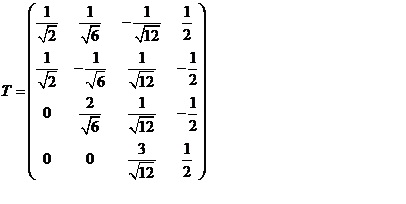.
而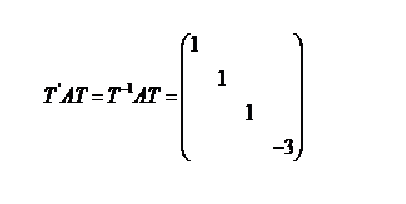.
三、matlab实现过程
(1)求特征多项式和特征值

clc
clear all
A=[0 1 1 -1;1 0 -1 1 ;1 -1 0 1;-1 1 1 0]
p=poly(A)
%  p =    1.0000         0   -6.0000    8.0000   -3.0000
p1=poly2str(p,’x’)
% p1 =   x^4 - 6 x^2 + 8 x - 3
p2=sym(‘x^4 - 6 x^2 + 8 x - 3’) %怎样引用前面的平p1
s1=solve(p2)   %查看 s1 =-3
1
1
1
（2）求1的特征向量
B=1*eye(4,4)-A
c=rref(B);

%  c =

%    1    -1    -1     1
%    0     0     0     0
%   0     0     0     0
%   0     0     0     0
%相当于 x1-x2-x3+x4=0
%4个未知数，一个方程，有三个自由变量，自行设置 x1=1 ,x2=1,x3=0,de x4=0
x1=1 ,x2=0,x3=1,de x4=0
x1=-1 ,x2=0,x3=0,de x4=1
%得一个基础解系
a1=[1 1 0 0];
a2=[1 0 1 0];
a3=[-1 0 0 1];
%对上面的3个向量正交化
b1=a1;
b2=a2-a2.*b1/sqrt(b1.*b1);
b3=a3-a3.*b1/sqrt(b1.*b1)-a3.*b2/sqrt(b2.*b2);
format rat   %分数显示
jie=[b1;b2;b3]’

%jie =

1.0000    0.5000   -0.0000
1.0000   -0.5000    1.0000
0    0.5000    1.0000
0   -0.5000    2.0000


jie(abs(jie)<0.0001)=0;
%单位化
format short
d1=jie(:,1)/norm(jie(:,1));
d2=jie(:,2)/norm(jie(:,2));
d3=jie(:,3)/norm(jie(:,3));

format rat   %分数显示

D=[d1,d2,d3]
format rat   %分数显示
D =

0.7071    0.5000   -0.0000
0.7071   -0.5000    0.4082
0    0.5000    0.4082
0   -0.5000    0.8165


% D’*D

ans =

1.0000    0.0000    0.2887
0.0000    1.0000   -0.4082
0.2887   -0.4082    1.0000


这时第1个列向量和第三个列向量内积不为0，有误差，难道前面的正交化过程有误？

尝试改进一下
b1=a1;
b2=a2 -(a2*b1’/(b1*b1’))*b1;
b3=a3-(a3*b1’/ (b1*b1’))*b1-(a3*b2’/ (b2*b2’))*b2;
format rat   %分数显示
jie=[b1;b2;b3]’
jie =

1.0000    0.5000   -0.3333
1.0000   -0.5000    0.3333
0    1.0000    0.3333
0         0    1.0000


d1=jie(:,1)/norm(jie(:,1));
d2=jie(:,2)/norm(jie(:,2));
d3=jie(:,3)/norm(jie(:,3));
D =

0.7071    0.4082   -0.2887
0.7071   -0.4082    0.2887
0    0.8165    0.2887
0         0    0.8660


D’*D

ans =

1.0000         0         0
0    1.0000   -0.0000
0   -0.0000    1.0000


（3）求-3的特征向量
B2=(-3)*eye(4,4)-A
C2=rref(B2)

%C2 =

 1     0     0    -1
0     1     0     1
0     0     1     1
0     0     0     0


有1个自由变量，取x1=1 得x4=1;x2=-1;x3=-1;
cc=[1 -1 -1 1]
cc=cc/norm(cc);

(4) T=[D,cc]
(5) T=[D,cc’]

T =

0.7071    0.4082   -0.2887   0.5
0.7071   -0.4082    0.2887    -0.5
0           0.8165     0.2887   -0.5
0             0              0.8660     0.5

T’*A*T
ans =

1.0000         0         0         0
0    1.0000   -0.0000         0
0         0    1.0000    0.0000
0         0         0   -3.0000


三 使用matlab函数分解

clc
clear all
A=[0 1 1 -1;1 0 -1 1 ;1 -1 0 1;-1 1 1 0]

[U,S,V]=svd(A,0)
U =

0.5000         0    0.8660   -0.0000


-0.5000   -0.0000    0.2887    0.8165
-0.5000    0.7071    0.2887   -0.4082
0.5000    0.7071   -0.2887    0.4082

S =

3.0000         0         0         0
0    1.0000         0         0
0         0    1.0000         0
0         0         0    1.0000


% 特征值前面是-3，在这是3；这个影响大不大？
V =

-0.5000         0    0.8660         0
0.5000         0    0.2887    0.8165
0.5000    0.7071    0.2887   -0.4082
-0.5000    0.7071   -0.2887    0.4082

[m,n] = size(A);
if m > 1, s = diag(S);
elseif m == 1, s = S(1);
else s = 0;
end
tol = max(m,n) * max(s) * eps(class(A));
r = sum(s > tol);
Q = U(:,1:r);
Q =

0.5000         0    0.8660   -0.0000


-0.5000   -0.0000    0.2887    0.8165
-0.5000    0.7071    0.2887   -0.4082
0.5000    0.7071   -0.2887    0.4082
Q’*Q

ans =

1.0000         0   -0.0000   -0.0000
0    1.0000         0   -0.0000


-0.0000         0    1.0000   -0.0000
-0.0000    -0.0000    -0.0000   1.0000
U*S*V’

ans =

0.0000    1.0000    1.0000   -1.0000
1.0000   -0.0000   -1.0000    1.0000
1.0000   -1.0000   -0.0000    1.0000


-1.0000    1.0000    1.0000   -0.0000
U-Q

ans =

 0     0     0     0
0     0     0     0
0     0     0     0
0     0     0     0


Q*S*Q’

ans =

1.5000   -0.5000   -0.5000    0.5000


-0.5000    1.5000    0.5000   -0.5000
-0.5000    0.5000    1.5000   -0.5000
0.5000   -0.5000   -0.5000    1.5000

Q*S*V’

ans =

0.0000    1.0000    1.0000   -1.0000
1.0000   -0.0000   -1.0000    1.0000
1.0000   -1.0000   -0.0000    1.0000


-1.0000    1.0000    1.0000   -0.0000
Q*S*V’= U*S*V’，需要注意的是，U和V虽然十分相似，但符号不同，所以U和V不能互相代替。
展开全文• ## 矩阵对角线求和

千次阅读 2019-11-01 12:44:09
求一个3×3矩阵对角线元素之和。 【输入形式】 依次输入9个整数 【输出形式】 输出主对角线元素之和 【样例输入】 3 7 1 4 7 9 4 5 4 【样例输出】 14 【样例说明】 主对角线上的数为：3 、7、4，和为14。 ...
【问题描述】
求一个3×3矩阵主对角线元素之和。

【输入形式】
依次输入9个整数

【输出形式】
输出主对角线元素之和

【样例输入】
3 7 1

4 7 9

4 5 4

【样例输出】
14

【样例说明】
主对角线上的数为：3 、7、4，和为14。

【评分标准】
矩阵中的数都是不超过1000的非负整数。

#include<iostream>
using namespace std;
int main()
{
int a;
for(int i=0;i<3;i++)
{
for(int x=0;x<3;x++)
{
cin>>a[i][x];
}
}
cout<<a+a+a;
return 0;
}



展开全文c++
• 根据台湾交通大学开放课程线性代数（莊重 特聘教授主讲）之授课内容整理的线性代数笔记，本文主要涉及教材第五章的内容，讨论特征值和特征向量的定义、矩阵（或算子）对角化有关的一些结论
一、可对角化、特征值和特征向量的定义Defs: 1) A linear operator T: V→V，on a finite-dimensional vector space V is called diagonalizable if ∃ an ordered basis β s.t.[T]β is a diagonal matrix.2) Let A ∈ Mn×n(F), then A is said to be diagonalizable if LA is diagonalizable. Here LA: Fn→Fn, LA(x)=Ax, x∈F。Defs: 1) Let T be a linear operator on a vector space V. If ∃ a 非零元素 v ∈ V and a scalar λ∈F s.t. T(v)=λv, then λ is an eigenvalue（特征值） of T and v is its corresponding eigenvector（特征向量）. 2) Let  A ∈ Mn×n(F), if ∃ a 非零元素 v ∈ Fn and a scalar λ∈F s.t. A(v)=λv, then λ is an eigenvalue of A and v is its associated eigenvectors.  因为T是线性的，所以一个特征值所对应的特征向量有无穷多个，当我们找到一个特征向量，将其乘以一个非零的scalar，所得之结果仍为特征向量。二、可对角化的充分必要条件Thm5.1：A linear operator T on a finite-dimensional vector space V is diagonalizable ⇔ ∃ an ordered basis β={v1, v2, ... , vn} for V, consisting of eigenvectors of T. In such case, let D=[T]β , then Djj is the eigenvalue corresponding to theeigenvector vj .Let A ∈ Mn×n(F), then A is diagonalizable ⇔ ∃ an ordered basis β={v1, v2, ... , vn} for Fn with vj  being eigenvalues.Assume T is diagonalizable. Let β' be be another ordered basis for V. Then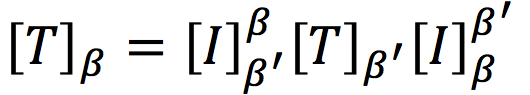. In particular, if T = LA and β' is the standard basis for Fn, then [T]β=Q-1AQ, where Q = (v1, v2, ... , vn). 三、特征值和特征向量的计算Thm5.2 Let A ∈ Mn×n(F), Then λ is an eigenvalue of A⇔det(A-λI)=0.Def: Let A ∈ Mn×n(F), Then f(λ) = det(A-λI) is called the characteristic polynomial (特征多项式) of A (eigenvalues 即是 A的特征多项式之零根).Let T be a linear operator on an n-dimensional vector space V with ordered basis β. We define the characteristic polynomial f(λ) of T to be the characteristic polynomial of A= [T]β. That is, f(λ) = det(A-λI).Thm5.4 v∈V is an eigenvector of T corresponding to λ ⇔ 非零元素 v 位于T -λI的零空间中，即 v∈N(T -λI) .四、可对角化的充分条件在Section2中，我们已经给出了可对角化的一个充要条件：一个线性算子（或一个矩阵）的特征值相对应的特征向量如果能构成向量空间的一个基底，那么这个线性算子（或矩阵）就是可对角化的。但是这个条件显然用起来并不那么方便，你要先算特征值，再计算对应的特征向量，然后还要检查特征向量是否线性独立。我们希望有一个更加简洁的条件来检验是否可对角化。为了得出这个更好用的条件，需要从下面这个Thm 5.5开始讨论。Thm 5.5 Let 是 λi T的特征值，vi是对应的特征向量。如果λi 各不相同，其中i = 1,2,...,k，那么{v1, v2, ... , vk}是线性独立的。（Hint：证明的方法主要是数学归纳法，这里不做详述）Remark：Thm 5.5的逆命题不成立。Thm 5.5说明一组各不相同的特征值所对应的特征向量彼此线性独立。但是如果有一组彼此线性独立的特征向量，其对应回去的特征值是否各不相同是无法保证的。一个简单的反例就是单位矩阵，例如 I = {1 0; 0 1}，当λ=1时，任何不为0的向量都是对应的特征向量，但这些彼此独立的特征向量对应的特征值都是1。推论：Let dim(V) = n, and T: V→V是线性的，或者A ∈ Mn×n(F)，如果T（或A）有n个不同的特征值，⇒ T（或A）是可对角线化的。这个推论是很显然的，因为T（或A）有n个不同的特征值，根据Thm5.5，它就有n个线性独立的特征向量。而dim(V) = n，所以这n个线性独立的特征向量就构成了一组基底。再由Thm5.1就可以得出T（或A）可对角线化的结论。当然，这个推论的逆命题仍然是不成立的，也就是说它不是可对角线化的充要条件。为了要得到一个更强的结论，我们还要讨论，如果特征多项式有重根，那么矩阵（或算子）还可以对角线化吗？五、几个重要的定义后面我们需要用到如下几个重要的定义。Def 1: A polynomial f(t) in P(F) splits over F, if f(t) can be factored as a product of the first degree polynomials in P(F)。F对于这个定义非常重要，必须要先指定。例如，F=R，那么(t2 + 1)(t - 2) does not split over R. However, it does split over C。Thm 5.6：T: F→F，或A ∈ Mn×n(F)，是可对角化的 ⇒ T（或A）的特征多项式splits over F。Def 2: Let f(t) and λ 分别是T的特征多项式和特征值。那么λ 的代数重数（algebraic multiplicity）是最大的正整数k for which (1-λ)k is the factor of f(t)。Def 3: 我们把Eλ = {v∈V: T(v) = λv} = {v∈V: (T - λI)(v) = 0} 定义为的eigenspace。也就是说Eλ 是个集合，这个集合收集了T的所有特征向量，以及零向量（把零向量也一同收进来的原因就在于使其形成一个子空间，这样才可以进一步去讨论维度的问题）。Remark: Eλ 是V的一个子空间。既然Eλ 是V的一个子空间，那么就可以定义它的维度。于是又有了如下定义。Def 4: 定义dim(Eλ) 是λ的几何重数（geometric multiplicity），记为gm(λ)。例如：对于单位矩阵 I = {1 0; 0 1}∈ M2×2(R)，它的特征多项式 f(t) = det(I-tI)=(1-t)2 ，所以λ = 1的am=2。另外，很容易发现任何二维向量都是2×2单位矩阵的特征向量（因为Ix=1x，x对于任意二维向量都成立），所以可以知道Eλ=1 = R2 ，即dim(Eλ=1)=2，所以λ = 1的gm=2。关于am和gm有如下一些结论（它在证明Section 6中的定理时会被用到）：1）(Thm 5.7) 1 ≤ gm(λ) ≤ am(λ)2）(Thm 5.8) Let λi be distinct eigenvalues of T, where i = 1, 2, ..., k. Let Si be a finite 线性独立 subset of eigenspace Eλi. Then S = S1∪S2∪...∪S3 是线性独立的。该定理的图形化解释如下图所示：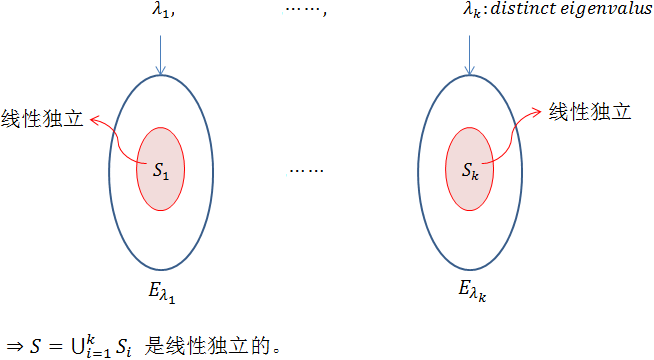六、可对角化的条件我们希望有一个更加简洁的条件来检验是否可对角化。而且是充分必要的条件。下面这个定理表明在某些条件下，只要检查特征值的一些情况就可以判断对角化的情况。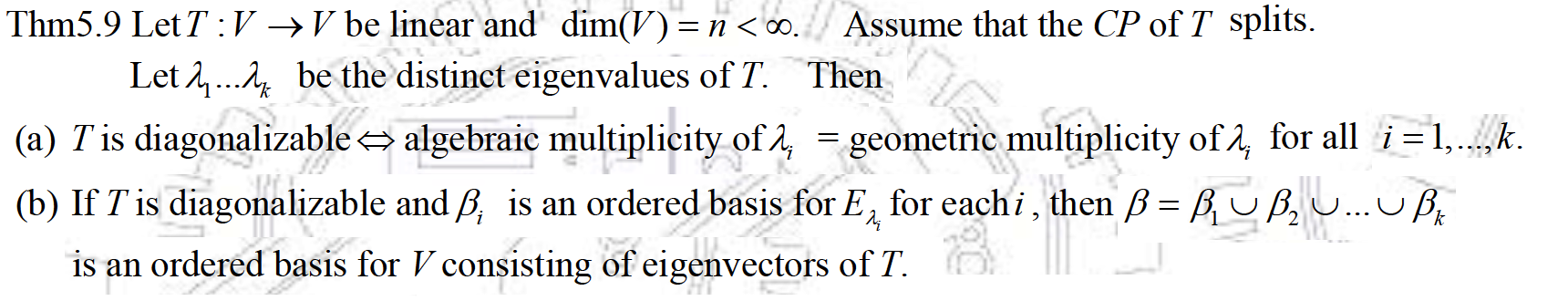注意k可以是小于等于n的，所以其实这定理是允许有重根的情况。这个定理的详细证明可以参考文献【1】。下面来看几个例子。首先，请问下面这个矩阵A可以对角化吗？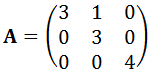为此要先来求它的特征多项式，即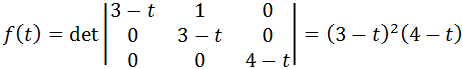可以看到它有两个不同的特征值，当λ = 4时 am =1 ，而且根据Thm 5.7可知其gm =1。当 λ = 3时 am =2 ，根据dimenstion theroy还可以算得此时gm = 1。由于定理的要求是所有的几何重数都等于代数重数，显然这里无法满足，所以矩阵A是不能对角化的。再来看一个算子的例子。T : P2(R)→P2(R), T(f(x))=f(1)+f'(0)x+(f'(0)+f''(0))x2.P2(R)→P2(R)表示线性算子T的作用是把一个二次多项式转换到一个二次多项式，符号P2(R)表示定义在R上的2次多项式。现在问T可否对角化？（应该说明对于一个算子T而言，要利用定理5.9就必须要先求出它的矩阵表示。如果选择的基底不同，那么对应的矩阵表示其实也会不同，但是最终的特征多项式并不会有影响，所以其实对于算子而言，如果要检查其可否对角化，那么大可以随便选择一个基底来求其矩阵表示）对于二次多项式来说，可以选择标准基底 β = {1, x, x2}，然后求算子T的矩阵表达，则有T(1) = 1, T(x) = 1+ x + x2, T(x2) = 1 + 2x2然后把系数依次摆成矩阵就得到了T的一个矩阵表示法，即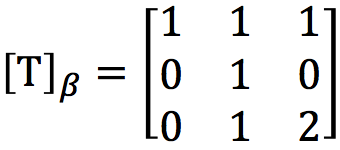在求出它的特征多项式为f(t) = (1-t)2(2-t), 于是可知当t=2时，am=gm=1，当t=1时，am=gm=2。所以根据Thm 5.9可知T是可对角线化的。既然算子T是可以对角线化的。根据定义其实就表明可以找到一个基底r，使得T的矩阵表示[T]r是一个对角线矩阵。上面我们使用的标准基底β所对应的矩阵表示不是一个对角矩阵。那么下面我们想问，如何找出那样一个基底r ？基本的找法可以总结为：由[T]β找Q，再找r。因为对于一个矩阵[T]β而言，说其实可以对角化的就意味着存在一个Q，使得Q-1[T]βQ=D，而D是一个对角线矩阵。根据定理5.1(3)可知首先要找的就是特征向量，然后由特征向量来把Q组出来。现在[T]β有两个特征值，当t=1时，其am=2，所以可以找出2个线性独立的特征向量，当t=2时，am=1，所以可以找出一个特征向量，用这三个向量就可以组成Q。当t=1时，就要先求[T]β-tI的零空间，即解下面这个方程组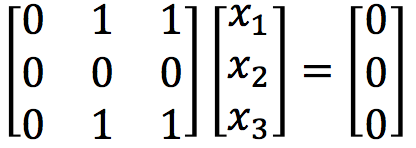解得x2 + x3  = 0, 所以只有写出满足这个条件的两个线性独立的向量即可，例如(1, 0, 0)T和(0, -1, 1)T。当t=2时，就要先求[T]β-tI的零空间，即解下面这个方程组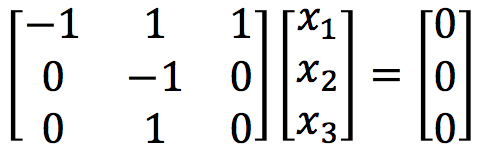解得-x1 + x2 + x3  = 0, x2 = 0, 所以可以写出向量为(1, 0, 1)T。把这三个向量合起来就得到了Q，即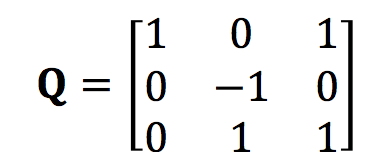这也就得到了r = {1,  -x + x2, 1+ x2}，用这个基底再来写T的矩阵表示，就会得到一个对角线矩阵。本文主要根据台湾交通大学开放课程线性代数（莊重 特聘教授主讲）之授课内容整理，并参考以下书籍：【1】S.H. Friedberg, A.J. Insel, L.E Spence, 4th edition, Linear Algebra, Prentice-Hall, 2003【2】David C. Lay.  刘深泉，等译. 线性代数及其应用（原书第3版），机械工业出版社，2005
展开全文特征向量
• ## CSS实现圆角矩阵

千次阅读 2014-04-11 18:25:58
） 其他浏览器对于前面解释的斜线（“/”）符号的支持各不相同，有些浏览器椭圆形（与圆形相对）边框提供有限的支持。 若要确保与旧版本浏览器的向后兼容性，您应参见相应的浏览器供应商网站。 根据浏览器和...css3 mozilla
• 对于3×33\times 3的例子，我们能够写出所有...我们现在引进矩阵符号来描述开始的系统，用矩阵乘法来描述计算步骤会更简单。注意三种不同类型的量都出现在例子中： Nine coefficientsThree unknownsThree right−h
• 文章目录Matlab 矩阵及其基本运算1、矩阵表示1.1数值矩阵的生成**1.2 符号矩阵的生成**1.3 大矩阵的生成1.4 多维数组的创建1.5 特殊矩阵的生成 Matlab 矩阵及其基本运算 Matlab 的功能非常强大，在计算领域是必备...matlab
• ## 符号矩阵（1）

千次阅读 2019-07-03 22:48:37
这时的函数sym实际上是在定义一个符号表达式，这时的符号矩阵中的元素可以是任何的符号或者表达式，而且长度没有限制，只是将方括号置于用于创建符号表达式的单引号中。 sym_martix = sym('[a,b,c;Jcak,HelpMe,NOWAY...
• 变换的矩阵表示形式 (原文)http://msdn.microsoft.com/zh-cn/library/vstudio/8667dchf.aspx m×n 矩阵是排列在m 行和 n 列中的一系列数。 下图显示几个矩阵。 您可以通过将单个元素相加来加合两个尺寸...
• 在MATLAB中利用符号函数功能，通过手动输入DH参数符号矩阵 or Excel文件导入DH参数表从而获得DH符号矩阵公式1.前言2.废话不多说，上代码演示效果1. **函数使用方法一：==通过excel直接导入DH参数获得DH矩阵==**2. **...matlab应用
• 表示是一种多多的关系的数据结构。因为线性表表示的是一种一一的关系的数据结构，树表示的是一种一多的数据结构，所以图把线性表和树都包含在内。图由一个非空的有限顶点集合和一个有限边集合组成。当我们...
• 取对角元素构成向量，或根据向量构成对角矩阵 [V,D]=eig(A) 特征值分解，使 AV=VD （没看懂） expm(A) 矩阵指数e^A inv(A) A的逆矩阵 [V,J]=jordan(A) Jordan分解，使AV=VJ poly(A) 矩阵的特征多项式 rankmatlab
• ## 向量的表示及协方差矩阵

万次阅读 多人点赞 2014-01-13 20:26:05
一般的，如果我们有M个N维向量，想将其变换为由R个N维向量表示的新空间中，那么首先将R个基按行组成矩阵A，然后将向量按列组成矩阵B，那么两矩阵的乘积AB就是变换结果，其中AB的第m列为A中第m列变换后的结果。...计算机视觉 图像分析 向量
• 本微信图文主要介绍了旋转矩阵、对角矩阵和矩阵乘法的几何与物理意义。旋转矩阵 正交矩阵 矩阵乘法
• 的右下注脚mn表示该方阵是由n坐标系变换到m坐标系的变换矩阵。方阵 C mn 中的每一个元素都是如表8.1.1所列 相应两轴夹角的余弦 ，称方向余弦，故该方阵称为 方向余弦矩阵 (orientation cosine matrix ) 。 相应两...
• 1.matlab中就是直接输出答案 >> 1+1 ans = 2 1*9 ans = ...2.matlab中输出矩阵 ...空格表示行，有分号表示换行 a=[1 2 3 4] a = 1 2 3 4 >> b=[1;2;3;4] b = 1 2 3 ...matlab 四则运算
• 矩阵对角化引入探讨线性变换的对角化问题。（定义、等价命题和定理）线性代数 线性变换
• 给定一个 n × n 的二维矩阵表示一个图像。 将图像顺时针旋转 90 度。 说明： 你必须在原地旋转图像，这意味着你需要直接修改输入的二维矩阵。请不要使用另一个矩阵来旋转图像。 示例 1: 给定 matrix = [ [1,2,3]...
• 再介绍奇异矩阵以及矩阵的广义逆之前，先复习几个概念，然后我一步步给出奇异矩阵和广义逆的介绍。 伴随矩阵 1. 余子式 定义1 余子式：在nnn阶行列式|A||A||A|中，划去元素aijaija_{ij}所在的行和列，剩下的...代数余子式
• 矩阵的正规性是检验矩阵是否可对角化的一个简便方法：任意正规矩阵都可在经过一个酉变换后变为对角矩阵，反过来所有可在经过一个酉变换后变为对角矩阵的矩阵都是正规矩阵。 在复系数矩阵中，所有的酉矩阵、埃尔matrix c 算法
•  下图是由14个“+”和14个“-”组成的符号三角形。2个同号下面都是“+”，2个异号下面都是“-”。  + + - + - + +  + - - - - +  - + + + -  - + + -  - + -  -dfs
• ## OpenCV—基本矩阵操作与示例

万次阅读 多人点赞 2016-05-02 16:30:14
图像处理中对矩阵的操作非常重要，本文总结了使用OpenCV进行矩阵的创建、初始化以及基本矩阵操作，给出了示例代码。OpenCV C++ 图像处理
• (F)）的内积为：,B> = tr(B*A)，其中tr表示矩阵的迹，也就是对角线上元素的和。 一些基于内积的重要定义（V is an inner product space）： Let x∈V, the length or norm of x is defined to be x, y ∈V is ...内积空间
• 矩阵A无逆矩阵,则称A为奇异矩阵。若A有逆矩阵,则称A是非奇异矩阵,简称非异阵 N阶行列式的超平行多面体的几何图形是由行(或列)向量张成的，而且这个n维超平行多面体与一个n维超长方体等体积。 一、代数意义 ...
• 如下图是由14个“+”和14个“-”组成的符号三角形, 2个同号下面都是“+”，2个异号下面都是“-”。  - + + - + + +   - + - - + +   - - + - +   + - - -   - + +   -算法
• 如果一个矩阵有n个线性无关的特征向量，则矩阵是可对角化的，矩阵表示成，相应的。因为，很有可能A的逆等于A的转置。同样的，就可能有，这可发现S中的特征向量和其他的特征向量正交，后文会进行证明。 我们把上的S...
• 一般的，如果我们有M个N维向量，想将其变换为由R个N维向量表示的新空间中，那么首先将R个基按行组成矩阵A，然后将向量按列组成矩阵B，那么两矩阵的乘积AB就是变换结果，其中AB的第m列为A中第m列变换后的结果。...PCA
• ## 矩阵行列式

千次阅读 2018-04-02 11:15:36
主要记录几个矩阵行列式容易忽略的性质 1.矩阵转置的行列式和原矩阵的行列式相等 2.交换矩阵的任意两行或者两列，行列式符号...5.上三角矩阵和下三角矩阵的行列式等于对角线元素的乘积 PS:矩阵是用word手写的...线性代数
• ## 矩阵

千次阅读 2012-09-09 11:15:21
矩阵 维基百科，自由的百科全书 线性代数 向量 · 矩阵 · 行列式 · 线性空间 显示▼向量 显示▼矩阵与行列式 显示...算法 图像处理 汇编
• 1.上三角矩阵/下三角矩阵，三对角矩阵，带状矩阵 2.Toeplitz矩阵，Hankel矩阵，Vandermonde矩阵 3.Z矩阵，M矩阵，H矩阵，对角占优阵，非负矩阵 4.对称矩阵，反对称矩阵，Hermite矩阵，反Hermite矩阵，正交矩阵，......